SBOS632 September   2015

UNLESS OTHERWISE NOTED, this document contains PRODUCTION DATA.

1. Features
2. Applications
3. Description
4. Revision History
5. Pin Configuration and Functions
6. Specifications
7. Detailed Description
1. 7.1 Overview
2. 7.2 Functional Block Diagram
3. 7.3 Feature Description
4. 7.4 Device Functional Modes
8. Application and Implementation
1. 8.1 Application Information
2. 8.2 Typical Application
9. Power Supply Recommendations
10. 10Layout
11. 11Device and Documentation Support
1. 11.1 Device Support
2. 11.2 Documentation Support
3. 11.3 Community Resources
5. 11.5 Electrostatic Discharge Caution
6. 11.6 Glossary
12. 12Mechanical, Packaging, and Orderable Information

• D|8
• DRJ|8
• DRJ|8

## 6 Specifications

### 6.1 Absolute Maximum Ratings

over operating free-air temperature range (unless otherwise noted)(1)
MIN MAX UNIT
Voltage Supply ±20 V
40 (single supply)
Current ±10 mA
Output short-circuit(3) Continuous
Temperature Operating range, TA –55 150 °C
Junction, TJ 150
Storage temperature, Tstg –65 150
(1) Stresses beyond those listed under Absolute Maximum Ratings may cause permanent damage to the device. These are stress ratings only, which do not imply functional operation of the device at these or any other conditions beyond those indicated under Recommended Operating Conditions. Exposure to absolute-maximum-rated conditions for extended periods may affect device reliability.
(2) Input pins are diode-clamped to the power-supply rails. Input signals that can swing more than 0.3 V beyond the supply rails must be current limited to 10 mA or less.
(3) Short-circuit to ground.

### 6.2 ESD Ratings

VALUE UNIT
V(ESD) Electrostatic discharge Human-body model (HBM), per ANSI/ESDA/JEDEC JS-001(1) ±2500 V
Charged-device model (CDM), per JEDEC specification JESD22-C101(2) ±1000
(1) JEDEC document JEP155 states that 500-V HBM allows safe manufacturing with a standard ESD control process.
(2) JEDEC document JEP157 states that 250-V CDM allows safe manufacturing with a standard ESD control process.

### 6.3 Recommended Operating Conditions

over operating free-air temperature range (unless otherwise noted)
MIN NOM MAX UNIT
VS Supply voltage 4 (±2) 36 (±18) V
Specified temperature -40 125 °C

### 6.4 Thermal Information

THERMAL METRIC(1) INA188 UNIT
D (SOIC) DRG (WSON)
8 PINS 8 PINS
RθJA Junction-to-ambient thermal resistance 125 145 °C/W
RθJC(top) Junction-to-case (top) thermal resistance 80 75 °C/W
RθJB Junction-to-board thermal resistance 68 39 °C/W
ψJT Junction-to-top characterization parameter 32 14 °C/W
ψJB Junction-to-board characterization parameter 68 105 °C/W
RθJC(bot) Junction-to-case (bottom) thermal resistance N/A N/A °C/W

### 6.5 Electrical Characteristics: VS = ±4V to ±18V (VS = 8V to 36V)

At TA = 25°C, RL = 10 kΩ, VREF = VS / 2, and G = 1, unless otherwise noted.
PARAMETER TEST CONDITIONS MIN TYP MAX UNIT
INPUT(1)
VOSI Input stage offset voltage At RTI(2) ±25 ±55 μV
At RTI, TA = –40°C to +125°C ±0.08 ±0.2 μV/°C
VOSO Output stage offset voltage At RTI ±60 ±170 μV
At RTI, TA = –40°C to +125°C ±0.2 ±0.35 μV/°C
VOS Offset voltage At RTI ±25 ±60 / G ±55 ±170 / G μV
At RTI, TA = –40°C to +125°C ±0.2 ±0.35 / G μV/°C
PSRR Power-supply rejection ratio G = 1, VS = 4 V to 36 V, VCM = VS / 2 ±0.7 ±2.25 µV/V
G = 10, VS = 4 V to 36 V, VCM = VS / 2 ±0.6
G = 100, VS = 4 V to 36 V, VCM = VS / 2 ±0.45
G = 1000, VS = 4 V to 36 V, VCM = VS / 2 ±0.3 ±0.8
Long-term stability 1(3) µV
Turn-on time to specified VOSI See the Typical Characteristics
zid Differential input impedance 100 || 6 GΩ || pF
zic Common-mode input impedance 100 || 9.5
VCM Common-mode voltage range The input signal common-mode range can be calculated with this tool (V–) + 0.1 (V+) – 1.5 V
CMRR Common-mode rejection ratio G = 1, at dc to 60 Hz, VCM = (V–) + 1.0 V to
(V+) – 2.5 V
84 90 dB
G = 10, at dc to 60 Hz, VCM = (V–) + 1.0 V to (V+) – 2.5 V 104 110
G = 100, at dc to 60 Hz, VCM = (V–) + 1.0 V to (V+) – 2.5 V 118 130
G = 1000, at dc to 60 Hz, VCM = (V–) + 1.0 V to (V+) – 2.5 V 118 130
INPUT BIAS CURRENT
IIB Input bias current ±850 ±2500 pA
TA = –40°C to +125°C See Figure 10 pA/°C
IOS Input offset current ±850 ±2500 pA
TA = –40°C to +125°C See Figure 11 pA/°C
INPUT VOLTAGE NOISE
eNI Input voltage noise f = 1 kHz, G = 100, RS = 0 Ω 12.5 nV/√Hz
f = 0.1 Hz to 10 Hz, G = 100, RS = 0 Ω 0.25 μVPP
eNO Output voltage noise f = 1 kHz, G = 100, RS = 0 Ω 118 nV/√Hz
f = 0.1 Hz to 10 Hz, G = 100, RS = 0 Ω 2.5 μVPP
iN Input current noise f = 1 kHz 440 fA/√Hz
f = 0.1 Hz to 10 Hz 10 pAPP
GAIN
G Gain equation 1 + (50 kΩ / RG) V/V
Gain range 1 1000 V/V
EG Gain error G = 1, (V–) + 0.5 V ≤ VO ≤ (V+) – 1.5 V ±0.007% ±0.025%
G = 10, (V–) + 0.5 V ≤ VO ≤ (V+) – 1.5 V ±0.05% ±0.20%
G = 100, (V–) + 0.5 V ≤ VO ≤ (V+) – 1.5 V ±0.06% ±0.20%
G = 1000, (V–) + 0.5 V ≤ VO ≤ (V+) – 1.5 V ±0.2% ±0.50%
Gain versus temperature G = 1, TA = –40°C to +125°C 1 5 ppm/°C
G > 1(4) , TA = –40°C to +125°C 15 50
Gain nonlinearity G = 1, VO = –10 V to +10 V 3 8 ppm
G > 1, VO = –10 V to +10 V See Figure 42 to Figure 45
OUTPUT
Output voltage swing from rail(5) RL = 10 kΩ(5) 220 250 mV
ISC Short-circuit current Continuous to common ±18 mA
FREQUENCY RESPONSE
BW Bandwidth, –3 dB G = 1 600 kHz
G = 10 95
G = 100 15
G = 1000 1.5
SR Slew rate G = 1, VS = ±18 V, VO = 10-V step 0.9 V/μs
G = 100, VS = ±18 V, VO = 10-V step 0.17
tS Settling time To 0.1% G = 1, VS = ±18 V, VSTEP = 10 V 50 μs
G = 100, VS = ±18 V, VSTEP = 10 V 400
To 0.01% G = 1, VS = ±18 V, VSTEP = 10 V 60 μs
G = 100, VS = ±18 V, VSTEP = 10 V 500
Overload recovery 50% overdrive 75 μs
REFERENCE INPUT
RIN Input impedance 40
Voltage range V– V+ V
POWER SUPPLY
Voltage range Single 4 36 V
Dual ±2 ±18
IQ Quiescent current VIN = VS / 2 1.4 1.6 mA
TA = –40°C to +125°C 1.8
TEMPERATURE RANGE
Specified temperature range –40 125 °C
Operating temperature range –55 150 °C
(1) Total VOS, referred-to-input = (VOSI) + (VOSO / G).
(2) RTI = Referred-to-input.
(3) 300-hour life test at 150°C demonstrated a randomly distributed variation of approximately 1 μV.
(4) Does not include effects of external resistor RG.
(5) See Typical Characteristics curves, Output Voltage Swing vs Output Current (Figure 19 to Figure 22).

### 6.6 Electrical Characteristics: VS = ±2V to < ±4V (VS = 4V to < 8V)

At TA = 25°C, RL = 10 kΩ, VREF = VS / 2, and G = 1, unless otherwise noted. Specifications not shown are identical to the Electrical Characteristics table for VS = ±2 V to ±18 V (VS = 8 V to 36 V).
PARAMETER TEST CONDITIONS MIN TYP MAX UNIT
INPUT(1)
VOSI Input stage offset voltage At RTI(2) ±25 ±55 μV
At RTI, TA = –40°C to +125°C ±0.08 ±0.2 μV/°C
VOSO Output stage offset voltage At RTI ±60 ±170 μV
At RTI, TA = –40°C to +125°C ±0.2 ±0.35 μV/°C
VOS Offset voltage At RTI ±25 ±60 / G ±55 ±170 / G μV
At RTI, TA = –40°C to +125°C ±0.2 ±0.35 / G μV/°C
Long-term stability 1(2) µV
Turn-on time to specified VOSI See the Typical Characteristics
zid Differential input impedance 100 || 6 GΩ || pF
zic Common-mode input impedance 100 || 9.5
VCM Common-mode voltage range VO = 0 V, the input signal common-mode range can be calculated with this tool (V–) (V+) – 1.5 V
CMRR Common-mode rejection ratio G = 1, at dc to 60 Hz, VCM = (V–) + 1.0 V to
(V+) – 2.5 V
80 90 dB
G = 10, at dc to 60 Hz, VCM = (V–) + 1.0 V to
(V+) – 2.5 V
94 110
G = 100, at dc to 60 Hz, VCM = (V–) + 1.0 V to (V+) – 2.5 V 102 120
G = 1000, at dc to 60 Hz, VCM = (V–) + 1.0 V to (V+) – 2.5 V 102 120
INPUT BIAS CURRENT
IIB Input bias current ±850 ±2500 pA
TA = –40°C to +125°C See Figure 10 pA/°C
IOS Input offset current ±850 ±2500 pA
TA = –40°C to +125°C See Figure 11 pA/°C
INPUT VOLTAGE NOISE
eNI Input voltage noise f = 1 kHz, G = 100, RS = 0 Ω 12.5 nV/√Hz
f = 0.1 Hz to 10 Hz, G = 100, RS = 0 Ω 0.25 μVPP
eNO Output voltage noise f = 1 kHz, G = 100, RS = 0 Ω 118 nV/√Hz
f = 0.1 Hz to 10 Hz, G = 100, RS = 0 Ω 2.5 μVPP
iN Input current noise f = 1 kHz 430 fA/√Hz
f = 0.1 Hz to 10 Hz 10 pAPP
GAIN
G Gain equation 1 + (50 kΩ / RG) V/V
Gain range 1 1000 V/V
EG Gain error G = 1, (V–) + 0.5 V ≤ VO ≤ (V+) – 1.5 V ±0.007% ±0.05%
G = 10, (V–) + 0.5 V ≤ VO ≤ (V+) – 1.5 V ±0.07% ±0.2%
G = 100, (V–) + 0.5 V ≤ VO ≤ (V+) – 1.5 V ±0.07% ±0.2%
G = 1000, (V–) + 0.5 V ≤ VO ≤ (V+) – 1.5 V ±0.25% ±0.5%
Gain versus temperature G = 1, TA = –40°C to +125°C 1 5 ppm/°C
G > 1(3), TA = –40°C to +125°C 15 50
Gain nonlinearity G = 1, VO = (V–) + 0.5 V ≤ VO ≤ (V+) – 1.5 V 3 8 ppm
OUTPUT
Output voltage swing from rail(5) RL = 10 kΩ 220 250 mV
ISC Short-circuit current Continuous to common ±18 mA
FREQUENCY RESPONSE
BW Bandwidth, –3 dB G = 1 600 kHz
G = 10 95
G = 100 15
G = 1000 1.5
SR Slew rate G = 1, VS = 5 V, VO = 4-V step 0.9 V/μs
G = 100, VS = 5 V, VO = 4-V step 0.17
tS Settling time To 0.1% G = 1, VS = 5 V, VSTEP = 4 V 50 μs
G = 100, VS = 5 V, VSTEP = 4 V 400
To 0.01% G = 1, VS = 5 V, VSTEP = 4 V 60 μs
G = 100, VS = 5 V, VSTEP = 4 V 500
Overload recovery 50% overdrive 75 μs
REFERENCE INPUT
RIN Input impedance 40
Voltage range V– V+ V
POWER SUPPLY
Voltage range Single 4 36 V
Dual ±2 ±18
IQ Quiescent current VIN = VS / 2 1.4 1.6 mA
TA = –40°C to +125°C 1.8
TEMPERATURE RANGE
Specified temperature range –40 125 °C
Operating temperature range –55 150 °C
(1) Total VOS, referred-to-input = (VOSI) + (VOSO / G).
(2) 300-hour life test at 150°C demonstrated randomly distributed variation of approximately 1 μV.
(3) Does not include effects of external resistor RG.

### 6.7 Typical Characteristics

At TA = 25°C, VS = ±15 V, RL = 10 kΩ, VREF = midsupply, and G = 1, unless otherwise noted.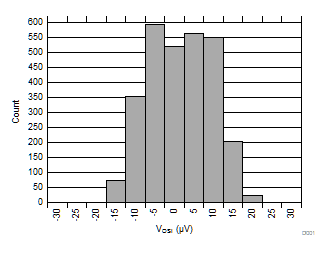Figure 1. Input Voltage Offset DistributionFigure 3. Output Voltage Offset Distribution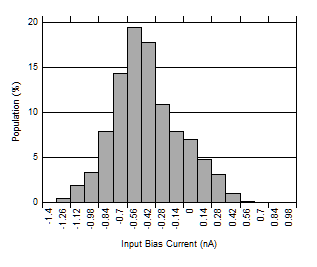Figure 5. Input Bias Current Distribution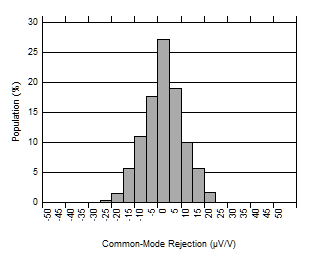G = 1
Figure 7. CMRR Distribution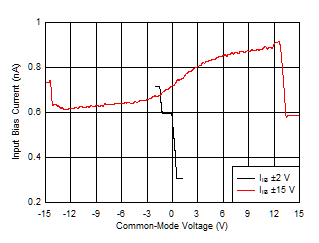Figure 9. Input Bias Current vs Common-Mode Voltage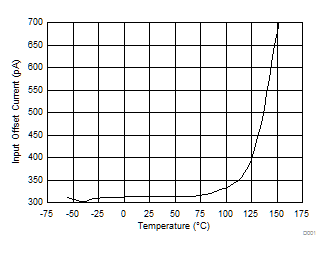Figure 11. Input Offset Current vs Temperature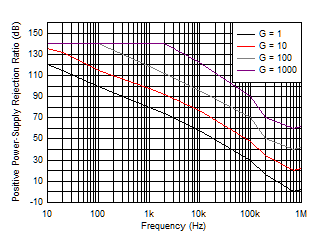At RTI
Figure 13. Positive PSRR vs FrequencyAt RTI
Figure 15. Gain vs Frequency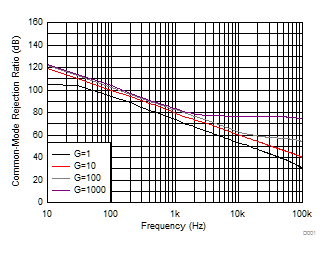At RTI, 1-kΩ Source Imbalance
Figure 17. CMRR vs Frequency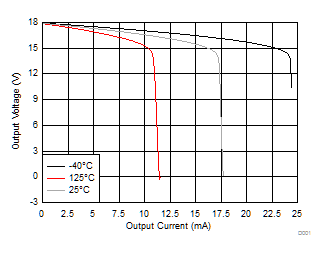VS = ±18 V
Figure 19. Positive Output Voltage Swing vs
Output Current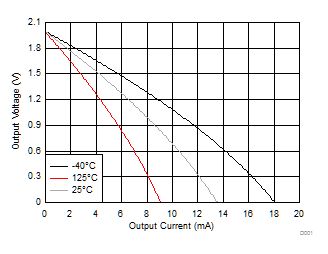VS = ±2 V
Figure 21. Positive Output Voltage Swing vs
Output Current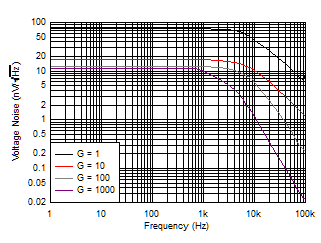Figure 23. Voltage Noise Spectral Density vs Frequency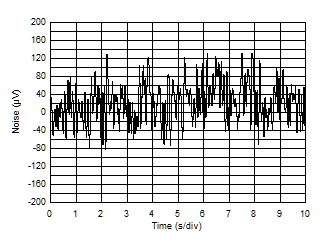G = 1000
Figure 25. 0.1-Hz to 10-Hz RTI Voltage Noise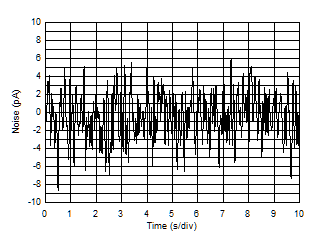Figure 27. 0.1-Hz to 10-Hz RTI Current Noise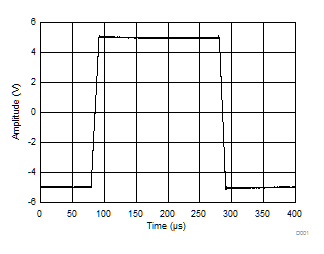RL = 10 kΩ, CL = 100 pF, G = 1
Figure 29. Large-Signal Pulse Response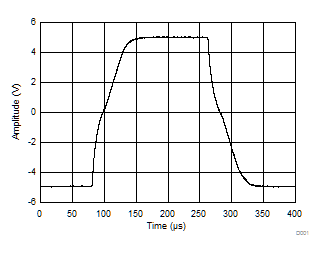RL = 10 kΩ, CL = 100 pF, G = 100
Figure 31. Large-Signal Pulse Response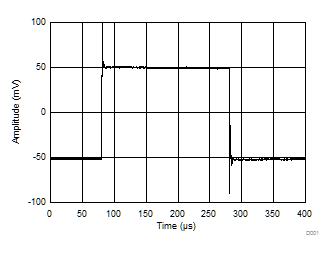RL = 10 kΩ, CL = 100 pF, G = 1
Figure 33. Small-Signal Pulse Response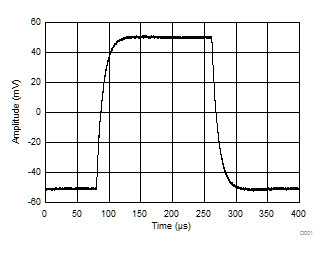RL = 10 kΩ, CL = 100 pF, G = 100
Figure 35. Small-Signal Pulse Response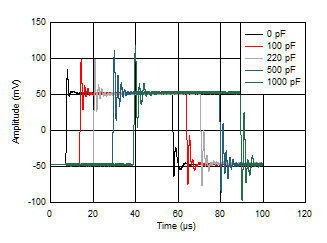G = 1
Figure 37. Small-Signal Response vs Capacitive Load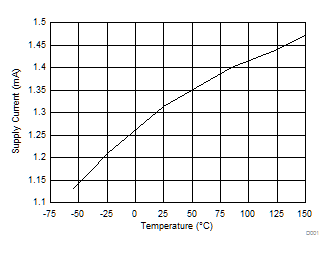Figure 39. Supply Current vs Temperature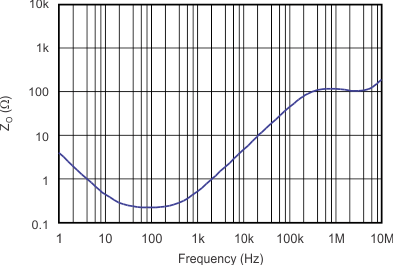Figure 41. Open-Loop Output Impedance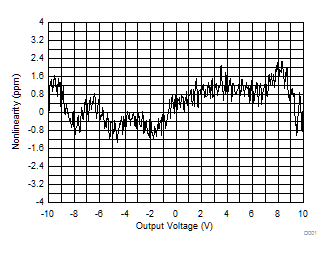G = 10
Figure 43. Gain Nonlinearity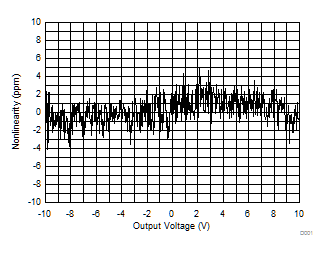G = 1000
Figure 45. Gain Nonlinearity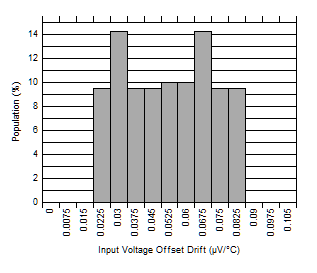–40°C to +125°C
Figure 2. Input Voltage Offset Drift Distribution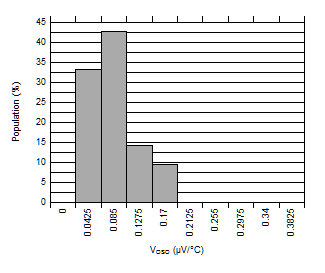–40°C to +125°C
Figure 4. Output Voltage Offset Drift Distribution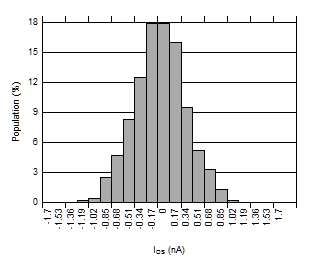Figure 6. Input Offset Current DistributionG = 100
Figure 8. CMRR Distribution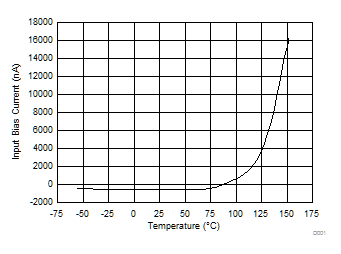Figure 10. Input Bias Current vs Temperature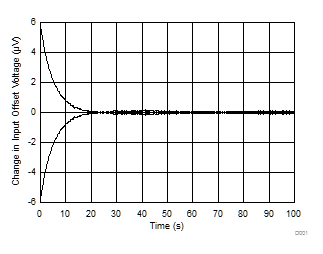Figure 12. Change in Input Offset Voltage vs Warm-Up Time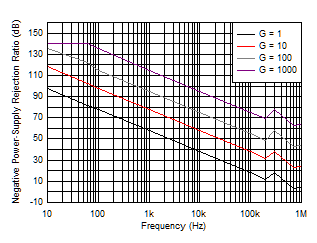Figure 14. Negative PSRR vs Frequency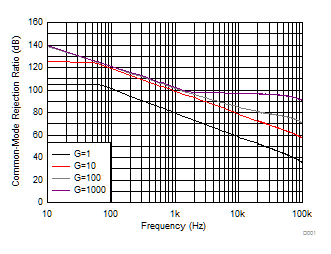At RTI
Figure 16. CMRR vs Frequency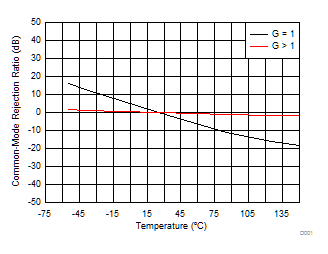Figure 18. Common-Mode Rejection Ratio vs Temperature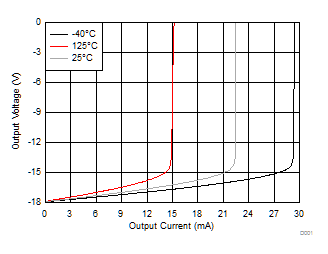VS = ±18 V
Figure 20. Negative Output Voltage Swing vs
Output Current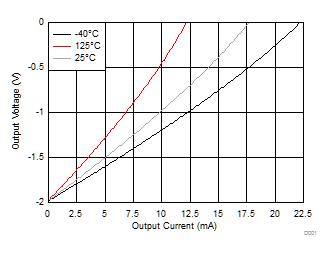VS = ±2 V
Figure 22. Negative Output Voltage Swing vs
Output Current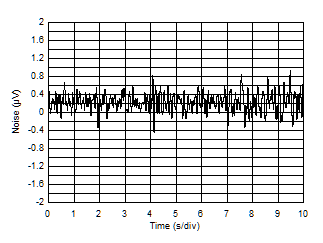G = 1
Figure 24. 0.1-Hz to 10-Hz RTI Voltage Noise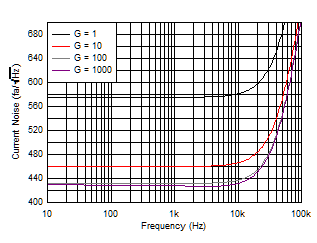Figure 26. Current Noise Spectral Density vs Frequency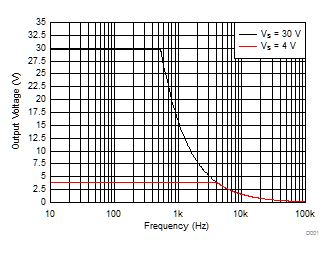Figure 28. Large-Signal Response vs Frequency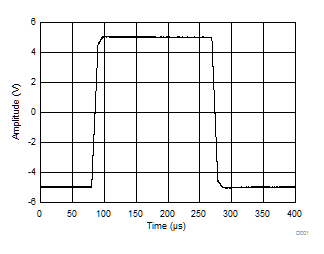RL = 10 kΩ, CL = 100 pF, G = 10
Figure 30. Large-Signal Pulse Response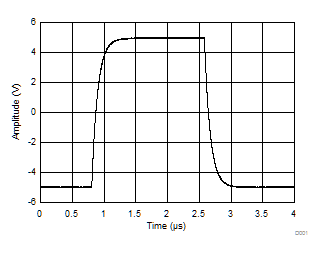RL = 10 kΩ, CL = 100 pF, G = 1000
Figure 32. Large-Signal Pulse Response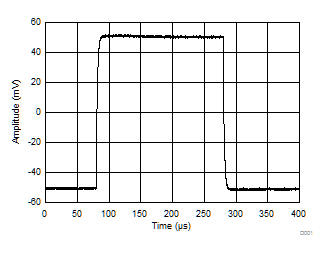RL = 10 kΩ, CL = 100 pF, G = 10
Figure 34. Small-Signal Pulse ResponseRL = 10 kΩ, CL = 100 pF, G = 1000
Figure 36. Small-Signal Pulse Response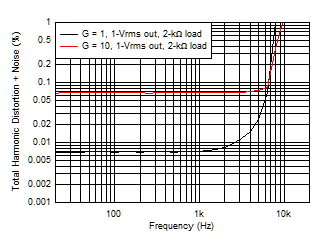Figure 38. Total Harmonic Distortion + Noise vs Frequency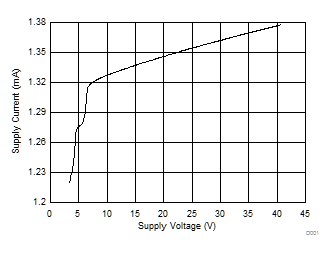Figure 40. Supply Current vs Supply Voltage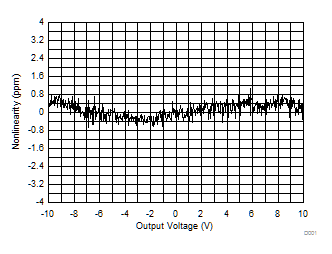G = 1
Figure 42. Gain Nonlinearity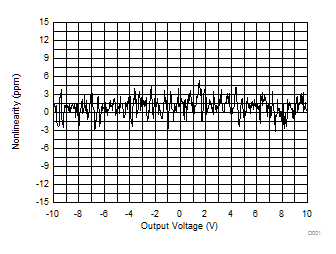G = 100
Figure 44. Gain Nonlinearity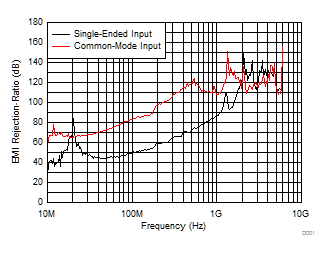Figure 46. EMIRR